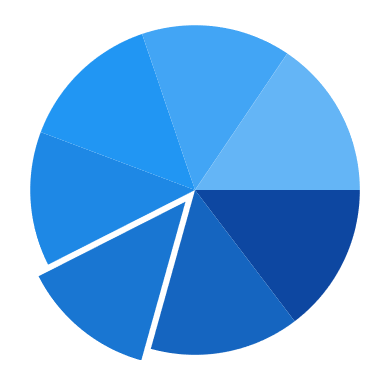# Explode segments in WinUI Chart (SfCircularChart)

13 Mar 20232 minutes to read

Exploding a segment is used to pull attention to a specific area of the circular chart. The following properties are used to explode the segments in the circular chart.

• ExplodeAll - Used to explode all the segments of these series.
• ExplodeIndex - Used to explode any specific segment.
• ExplodeRadius - Used to define the explode distance.
• ExplodeOnTap - Used to explode the segment when segment is clicked.

Explode Index

``````<chart:SfCircularChart>
. . .
<chart:PieSeries x:Name="PieSeries"
ItemsSource="{Binding Data}"
ExplodeIndex="2"
XBindingPath="Utilization"
YBindingPath="ResponseTime" />

</chart:SfCircularChart>``````
``````SfCircularChart chart = new SfCircularChart();
. . .
PieSeries series = new PieSeries()
{
ItemsSource = new ViewModel().Data,
XBindingPath = "Utilization",
YBindingPath = "ResponseTime",
ExplodeIndex = 2,
};NOTE

By default ExplodeRadius value is zero. So you need to define ExplodeRadius value, when you set ExplodeIndex or ExplodeAll.

Explode All

``````<chart:SfCircularChart>
. . .
<chart:PieSeries ExplodeAll="True"
XBindingPath="Category"
ItemsSource="{Binding Data}"
YBindingPath="Value">

</chart:SfCircularChart>``````
``````SfCircularChart chart = new SfCircularChart();
. . .
PieSeries series = new PieSeries()
{
ItemsSource = new ViewModel().Data,
XBindingPath = "Category",
YBindingPath = "Value",
ExplodeAll = true,